# 2015PUPC普林斯顿大学物理竞赛Onsite答案免费下载

### Onsite现场竞赛部分### 2015PUPC普林斯顿大学物理竞赛完整版真题免费下载

Onsite现场竞赛部分此卷共4题

### 2015PUPC普林斯顿大学物理竞赛完整版答案免费下载

Question 1

a. The initial energy isand the final energy is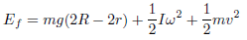For the ball not to lose contact,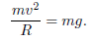Using conservation of energy, it follows that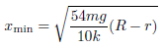b. A simple application of conservation of energy yields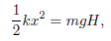or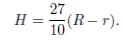Problem 2

Note that the moment of inertia of a uniformly distributed triangle with mass M and distance from center to vertex L is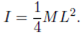a.  We apply conservation of angular momentum (about the center of the triangle), linear momentum, and energy. This gives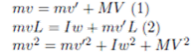Subtracting L times (1) from (2) gives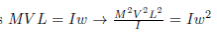which we can substitute to get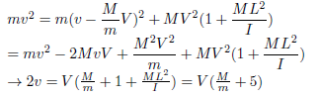###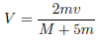Substituting into (1) gives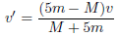Substituting into (2) gives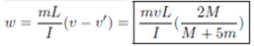b. In order for a second collision to occur, we need to consider v/ relative to V (i.e. consider the motion of the point mass in the frame of the triangle). Clearly if v/ >V , there will be no second collision since the point mass will ’escape’ the triangle’s reach -to see this clearly, draw the circle centered at the triangle’s center (i.e. the circumcircle of the triangle). The only possible point of contact for the second collision is at the bottom of this circle so the point mass must remain stationary relative to the triangle so that the triangle can rotate and collide with the point mass a second time.

Similarly, if v/ <V , then there will be no second collision.

If v'= V , then indeed there will be a second collision since this means the point mass remains stationary relative to the triangle while the triangle will spin with angular velocity w> 0.

Thus, a second collision occurs if V = v/ or 2m =5m − M

M=3m